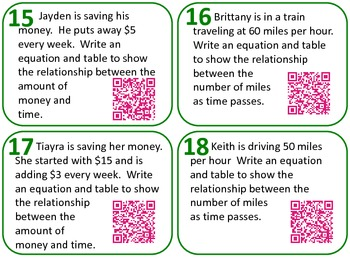QR Code Task Cards Grade 6 Expressions & Equations 2 Common Core AlignedSubject
Resource Type
Product Rating
File Type

PDF (Acrobat) Document File

Be sure that you have an application to open this file type before downloading and/or purchasing.

241 KB|18 cards
Share
Product Description
This set of task cards covers the last five skills of the Expressions and Equations Grade 6 Common Core.

• 6.EE.5-8 Reason about and solve one-variable equations and inequalities.
• 6.EE.9 Represent and analyze quantitative relationships between dependent and independent variables.

5. Understand solving an equation or inequality as a process of
answering a question: which values from a specified set, if any, make the equation or inequality true? Use substitution to determine whether a given number in a specified set makes an equation or inequality true.
6. Use variables to represent numbers and write expressions when
solving a real-world or mathematical problem; understand that a
variable can represent an unknown number, or, depending on the
purpose at hand, any number in a specified set.
7. Solve real-world and mathematical problems by writing and solving equations of the form x + p = q and px = q for cases in which p, q and x are all nonnegative rational numbers.
8. Write an inequality of the form x > c or x < c to represent a constraint or condition in a real-world or mathematical problem. Recognize that inequalities of the form x > c or x < c have infinitely many solutions; represent solutions of such inequalities on number line diagrams.

Represent and analyze quantitative relationships between
dependent and independent variables.

9. Use variables to represent two quantities in a real-world problem that change in relationship to one another; write an equation to express one quantity, thought of as the dependent variable, in terms of the other quantity, thought of as the independent variable. Analyze the relationship between the dependent and independent variables using graphs and tables, and relate these to the equation.
Total Pages
18 cards
Included
Teaching Duration
N/A
Report this Resource to TpT
Reported resources will be reviewed by our team. Report this resource to let us know if this resource violates TpT’s content guidelines.